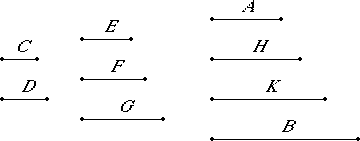# Proposition 15

If a cubic number measures a cubic number, then the side also measures the side; and, if the side measures the side, then the cube also measures the cube.

Let the cubic number A measure the cube B, and let C be the side of A and D the side of B.

I say that C measures D.

Multiply C by itself to make E, multiply D by itself to make G, multiply C by D to make F, and multiply C and D by F to make H and K respectively.Now it is clear that E, F, and G and A, H, K, and B are continuously proportional in the ratio of C to D. And, since A, H, K, and B are continuously proportional, and A measures B and G therefore it also measures H.

VII.Def.20

And A is to H as C is to D, therefore C also measures D.

Next, let C measure D.

I say that A also measures B.

VII.Def.20

With the same construction, we can prove in a similar manner that A, H, K, and B are continuously proportional in the ratio of C to D. And, since C measures D, and C is to D as A is to H, therefore A also measures H, so that A measures B also.

Therefore, if a cubic number measures a cubic number, then the side also measures the side; and, if the side measures the side, then the cube also measures the cube.

Q.E.D.

## Guide

c3 divides d3 if and only if c divides d.

This proposition is used to prove its contrapositive, VIII.17.